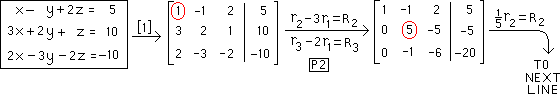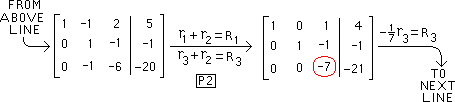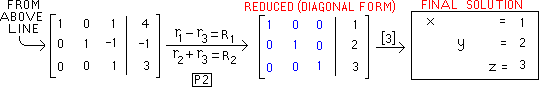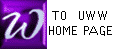The Gauss-Jordan Method Row-reduced formJ. Gauss:
1777-1855
M. Jordan:
1838-1922

GAUSS / JORDAN (G / J) is a device to solve systems of (linear) equations.
Given a system of equations, a solution using G / J follows these steps:


 Write the given system as an augmented matrix.
Examples of this step are below, or in specialized example "b",
or in specialized example "c", or in our text Rolf (Pg 88).

[system] ===> [ A | B ]

 Convert [ A | B ] to REDUCED FORM:
Pivot on matrix elements in positions 1-1, 2-2, 3-3, and so forth as far as is possible, in that order, with the objective of creating the biggest possible identity matrix I in the left portion of the augmented matrix. If one of these pivoting elements is zero, then first interchange it's row with a lower row. For all problems you will see this semester, this step  is equivalent to steps 1 through 7 on Pg 95-96 of Rolf, resulting in REDUCED FORM.

[ A | B ] ===> [ I | C ]

 When  is done, re-write the final matrix [ I | C ] as equations.
C will be a (vertical) list of variable values which solve the system, as in the example below

 Note 1: It is possible to vary the GAUSS/JORDAN method and still arrive at correct solutions to problems. For example, the pivot elements in step  might be different from 1-1, 2-2, 3-3, etc. Also, it is possible to use row operations which are not strictly part of the pivoting process. Students are nevertheless encouraged to use the above steps .

 Note 2: Professor McFarland names row operations just a bit differently from our text: follow Prof McFarland's naming style.

Note 3: Compare the steps of G / J with those for finding < a href="inverse.htm">matrix inverses.

 One simple example of G/J row operations is offered immediately above the pivoting reference; an example is below:
 Below is a system of equations which we will solve using G/J
 Below is the 1st augmented matrix : pivot on the "1" encircled in red
 Row operations for the 1st pivoting are named below
 Next we pivot on the number "5" in the 2-2 position, encircled belowBelow is the result of performing P1 on the element in the 2-2 position. Next we must perform P2
 Row operations of P2 are below
 The result of the 2nd pivoting is below. Now pivot on "-7" encircled in red
 Using P1 below we change "-7" to "1"Below is the result of performing P1 on "-7" in the 3-3 position. Next we must perform P2
 Row operations of P2 are below
 The result of the third (and last) pivoting is below with 3x3 ISM matrix in blue
 Step  of G/J
 Re-writing the final matrix as equations gives the solution to the original systemTo check your pivot calculations, try the PIVOT ENGINE.